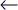# How to Calculate Flow Rate and Pressure Requirements for an Effective Slurry PumpBACK TO INSIGHT
02/2023

In order to choose a slurry pump that will operate successfully and efficiently, it is important to carefully analyze the flow rate and pressure requirements. In this post, we'll go through the main considerations that must be made when determining the flow rate and pressure specifications for a slurry pump.

## How to Calculate Flow Rate:

When choosing a slurry pump, the flow rate—or volume of slurry that needs to be pumped—must be taken into account. Gallons per minute (gpm) or cubic meters per hour (m3/hr) are common units used to express flow rates. You must be aware of the following factors in order to determine the flow rate precisely:

1. Slurry volume total: This is the total volume of slurry that must be pumped, and it is commonly expressed in cubic meters or gallons.

1. Slurry density: The mass of the slurry per unit volume, usually expressed in kg/m3 or lb/gal, is known as the slurry's density.

1. Solids content: The amount of solid particles by weight in a slurry is referred to as its solids content. Usually, a percentage is used to express this.

The time needed to pump the total volume of slurry is known as the required pumping time.

Once you have these variables, you can figure out the flow rate using the formula below:

Total Volume of Slurry (Gallons) x Pumping Time (Minutes) = Flow Rate (gpm).

## How to calculate pressure:

The distance the slurry needs to be pushed, the difference in elevation between the pump's inlet and exit, the viscosity of the slurry, and the pipe diameter all affect the pressure that a slurry pump needs to operate. In most cases, the pressure is expressed in terms of pounds per square inch (psi) or bars.

##### You will need to be aware of the following factors in order to calculate the pressure requirement:

1. Total head loss resulting from friction, elevation changes, and other causes is known as the total dynamic head. Typically, it is expressed in feet or meters.

1. Pipe diameter: The pipe's diameter will have an impact on the amount of pressure needed to transport the slurry. Usually, it is expressed in millimeters or inches.

1. Density and viscosity of the slurry: These factors will also affect the pressure needed to pump the slurry.

Once you have these variables, you may use the formula below to get the necessary pressure:

Elevation Pressure (psi) + Pipe Friction Loss (psi) + Total Dynamic Head (feet) x 0.433 = Pressure (psi).

To guarantee optimum performance, it is important to carefully evaluate the flow rate and pressure requirements while choosing a slurry pump. By precisely calculating these variables and accounting for other crucial elements, you may choose a pump that will satisfy your unique needs and offer dependable and effective operation.